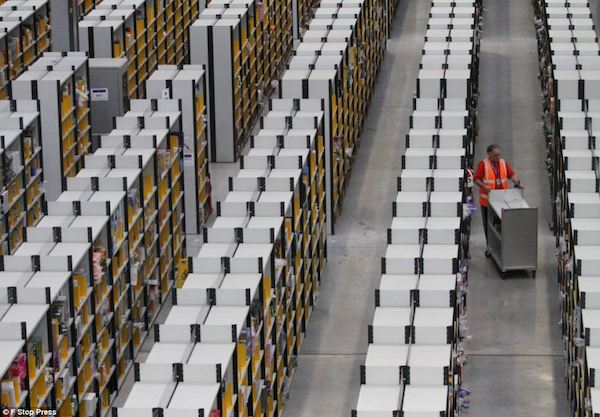# Houston, we've had a product here

If we take the product of all natural numbers $a$ and their inverses $a^{-1}$, we get 1, because we can use the one-to-one map $\displaystyle a\rightarrow a^{-1}$ and therefore\begin{aligned} \text{P } &=1\times\frac22 \times \frac33 \times \frac44 \times \cdots \\ &=\prod\limits_{a\in\mathbb{N}} aa^{-1} \\ &=\prod\limits_{a\in\mathbb{N}}1\\ &=1. \end{aligned}

If instead, we use the one-to-one map $a\rightarrow \frac{1}{a-1}$, the product seems to be larger than 1, because every term in the product is greater than 1:

\begin{aligned} \text{P } &= 1\times 2 \times \left(\frac32 \times \frac43 \times \frac54\right) \times \cdots\\ \displaystyle &= 2\prod\limits_{a>2\in\mathbb{N}} \frac{a}{a-1} \\ &= \infty. \end{aligned}

Finally, if we pick $a\rightarrow \frac{a}{a+1},$ the product seems to be zero.

Something is wrong. What's the deal?

×